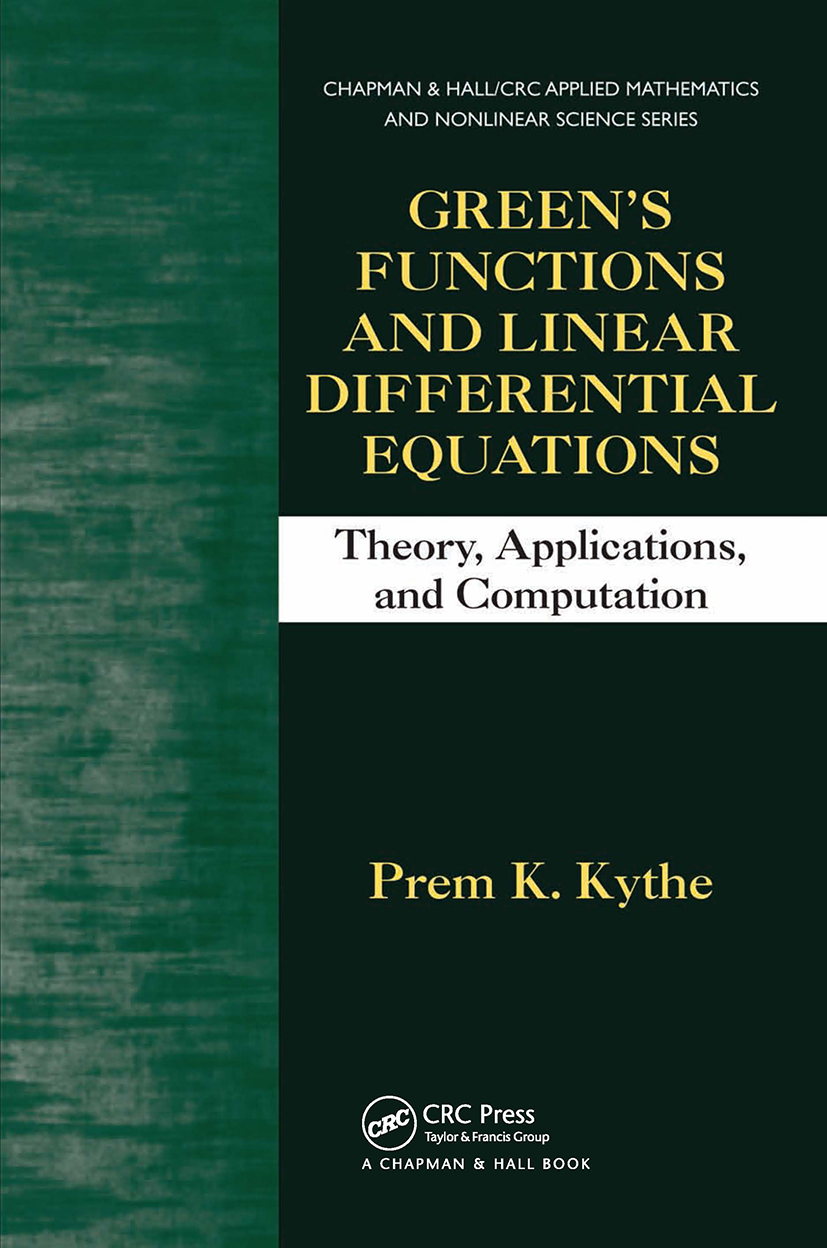# Green's Functions and Linear Differential Equations

## Theory, Applications, and Computation, 1st Edition

Chapman and Hall/CRC

382 pages | 47 B/W Illus.

##### Purchasing Options:\$ = USD
Hardback: 9781439840085
pub: 2011-01-21
\$155.00
x
eBook (VitalSource) : 9780429106439
pub: 2011-01-21
from \$28.98

FREE Standard Shipping!

### Description

Green’s Functions and Linear Differential Equations: Theory, Applications, and Computation presents a variety of methods to solve linear ordinary differential equations (ODEs) and partial differential equations (PDEs). The text provides a sufficient theoretical basis to understand Green’s function method, which is used to solve initial and boundary value problems involving linear ODEs and PDEs. It also contains a large number of examples and exercises from diverse areas of mathematics, applied science, and engineering.

Taking a direct approach, the book first unravels the mystery of the Dirac delta function and then explains its relationship to Green’s functions. The remainder of the text explores the development of Green’s functions and their use in solving linear ODEs and PDEs. The author discusses how to apply various approaches to solve initial and boundary value problems, including classical and general variations of parameters, Wronskian method, Bernoulli’s separation method, integral transform method, method of images, conformal mapping method, and interpolation method. He also covers applications of Green’s functions, including spherical and surface harmonics.

Filled with worked examples and exercises, this robust, self-contained text fully explains the differential equation problems, includes graphical representations where necessary, and provides relevant background material. It is mathematically rigorous yet accessible enough for readers to grasp the beauty and power of the subject.

### Table of Contents

Some Basic Results

Euclidean Space

Classes of Continuous Functions

Convergence

Functionals

Linear Transformations

Cramer’s Rule

Green’s Identities

Differentiation and Integration

Inequalities

The Concept of Green’s Functions

Generalized Functions

Singular Distributions

The Concept of Green’s Functions

Linear Operators and Inverse Operators

Fundamental Solutions

Sturm–Liouville Systems

Ordinary Differential Equations

Initial Value Problems

Boundary Value Problems

Eigenvalue Problem for Sturm–Liouville Systems

Periodic Sturm–Liouville Systems

Singular Sturm–Liouville Systems

Bernoulli’s Separation Method

Coordinate Systems

Partial Differential Equations

Bernoulli’s Separation Method

Examples

Integral Transforms

Integral Transform Pairs

Laplace Transform

Fourier Integral Theorems

Fourier Sine and Cosine Transforms

Finite Fourier Transforms

Multiple Transforms

Hankel Transforms

Summary: Variables of Transforms

Parabolic Equations

1-D Diffusion Equation

2-D Diffusion Equation

3-D Diffusion Equation

Schrödinger Diffusion Operator

Min-Max Principle

Diffusion Equation in a Finite Medium

Axisymmetric Diffusion Equation

1-D Heat Conduction Problem

Stefan Problem

1-D Fractional Diffusion Equation

1-D Fractional Schrödinger Diffusion Equation

Eigenpairs and Dirac Delta Function

Hyperbolic Equations

1-D Wave Equation

2-D Wave Equation

3-D Wave Equation

2-D Axisymmetric Wave Equation

Vibrations of a Circular Membrane

3-D Wave Equation in a Cube

Schrödinger Wave Equation

Hydrogen Atom

1-D Fractional Nonhomogeneous Wave Equation

Applications of the Wave Operator

Laplace Transform Method

Quasioptics and Diffraction

Elliptic Equations

Green’s Function for 2-D Laplace’s Equation

2-D Laplace’s Equation in a Rectangle

Green’s Function for 3-D Laplace’s Equation

Harmonic Functions

2-D Helmholtz’s Equation

Green’s Function for 3-D Helmholtz’s Equation

2-D Poisson’s Equation in a Circle

Method for Green’s Function in a Rectangle

Poisson’s Equation in a Cube

Laplace’s Equation in a Sphere

Poisson’s Equation and Green’s Function in a Sphere

Applications of Elliptic Equations

Spherical Harmonics

Historical Sketch

Laplace’s Solid Spherical Harmonics

Surface Spherical Harmonics

Conformal Mapping Method

Definitions and Theorems

Dirichlet Problem

Neumann Problem

Green’s and Neumann’s Functions

Computation of Green’s Functions

Appendix A: Adjoint Operators

Appendix B: List of Fundamental Solutions

Appendix C: List of Spherical Harmonics

Appendix D: Tables of Integral Transforms

Appendix E: Fractional Derivatives

Appendix F: Systems of Ordinary Differential Equations

Bibliography

Index

Exercises appear at the end of each chapter, with hints, answers, and, sometimes, complete solutions.

### About the Author

Prem K. Kythe is a professor emeritus of mathematics at the University of New Orleans. Dr. Kythe is the co-author of Handbook of Computational Methods for Integration (CRC Press, December 2004) and Partial Differential Equations and Boundary Value Problems with Mathematica, Second Edition (CRC Press, November 2002). His research encompasses complex function theory, boundary value problems, wave structure, and integral transforms.

### Subject Categories

##### BISAC Subject Codes/Headings:
MAT003000
MATHEMATICS / Applied
MAT004000
MATHEMATICS / Arithmetic
MAT007000
MATHEMATICS / Differential Equations
SCI040000
SCIENCE / Mathematical Physics